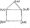# Computer Graphics and Visualization June 2010

PART-A

1. a  Discuss the applications of computer graphics.

b.  Giving the block diagram, explain the high-level view of a graphics system.

c.   Explain the different graphics architectures,

d.  Write a fragment of a simple program in pen plotter model that would generate the output shown inFig.Ql(d),Fig. Q 1(d)

2 a. Write a typical main function that works for moot noil-interactive applications and explain eachfimction call in it.

b.  Explain the major groups of graphics functions.

c.   Differentiate additive color model from subtractive color model.

3 a. What are the major characteristics that describe the logical behavior of an input device? Explain how open GL provides the functionality of each of the classes of logical input devices.

b.  What is a display list? Give the open GL code segment that generates a display list defining a red triangle with vertices at (50, 50), (150, 50) and (100, 150).

c.   What is double buffering? How is it implemented in open GL?

4 a. Explain the different open GL frames.

b.  Explain bilinear interpolation method of assigning colors to points inside a quadrilateral.

c.   Explain the properties that ensure that a polygon will be displayed correctly.

d.  Explain the following:

ii) Homogeneous coordinates.

PART-B

5 a. Explain the basic transformations in 3D and represent them in matrix form.

b.  What are the entities required to perform a rotation? Show that two rotations about the same axis commute.

c.   What is concatenation? How does it affect the efficiency of transformations?

d.  What are the advantages of quaternion’s?

6  a. Bring out the differences between perspective and parallel projections.

b.   Derive the simple perspective projection matrix.

c.   What is projection normalization?

d.   Explain the z – buffer algorithm giving its pseudo code.

7  a. What are the various methods available for shading a polygonal mesh?

b. Write the open GL code segment to approximate a sphere using subdivision. C. Explain the different types of light sources supported by open GL.

8 a. Use the Liang Barsky line – clipping algorithm to clip the line Pi(-15, -30) to P2(30, 60) against the window having diagonally opposite comers at (0, 0) and (15, 15).

b. Digitize the line from (10,16) to (16, 12) using the DDA algorithm.

c. Clip the polygon given in Fig.Q8(c) using the Sutherland Hodgeman polygon clipping algorithm.